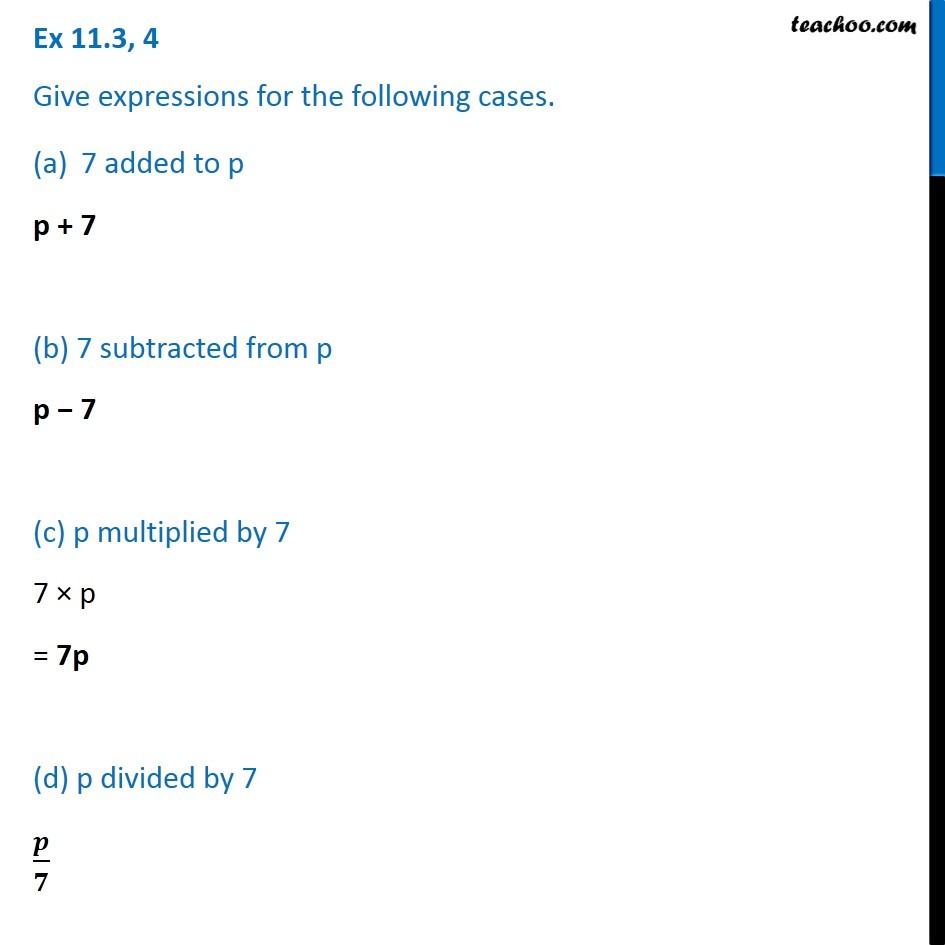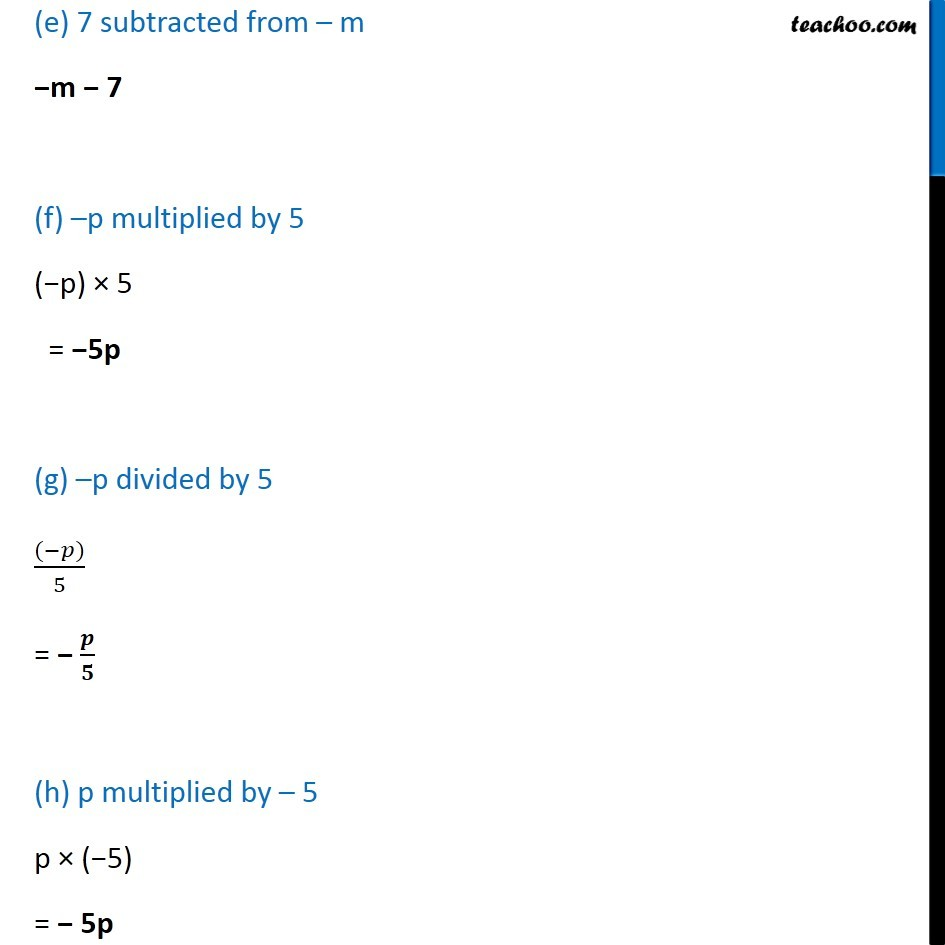Expression with variables

Chapter 11 Class 6 Algebra
Concept wiseLearn in your speed, with individual attention - Teachoo Maths 1-on-1 Class

### Transcript

Question 4 Give expressions for the following cases. 7 added to p p + 7 (b) 7 subtracted from p p − 7 (c) p multiplied by 7 7 × p = 7p (d) p divided by 7 𝒑/𝟕 (e) 7 subtracted from – m −m − 7 (f) –p multiplied by 5 (−p) × 5 = −5p (g) –p divided by 5 ((−𝑝))/5 = − 𝒑/𝟓 (h) p multiplied by – 5 p × (−5) = − 5p